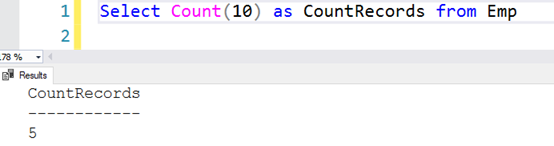top of page

# Difference between SQL COUNT(*), COUNT(1), COUNT(column_name) In SQL SERVER

Updated: Mar 29, 2022

What is the difference between COUNT(*), COUNT(1), and COUNT(column_name) in SQL Server? Read all about it here.

The SQL COUNT() function in SQL Server counts the number of rows and accepts only one argument. Although it is quite a simple function, still, it creates confusion with different argument values. For example, you might see T-SQL code using COUNT(*) or COUNT(1) or COUNT(Column_name) or COUNT(DISTINCT(Column_name).

What is a difference in query output with different arguments in SQL Server? Let’s find it out.

For the demonstration purpose, I have the following [Emp] table.

```Create table Emp
(
ID int identity(1,1) primary key,
[FirstName] varchar(20),
[MiddleName] varchar(20),
[LastName] varchar(20)
)
go
Insert into Emp([FirstName],[MiddleName],[LastName]) values ('Rajendra',NULL,'Gupta')
Insert into Emp([FirstName],[MiddleName],[LastName]) values ('Mohan','K','Sharma')
Insert into Emp([FirstName],[MiddleName],[LastName]) values ('Johan','L','Cruyff')
Insert into Emp([FirstName],[MiddleName],[LastName]) values ('Shyam','Sunder','Agarwal')
Insert into Emp([FirstName],[MiddleName],[LastName]) values ('Ranjith','M','Eswaran')
go
select * from Emp```The records show that all Employees except row 1 have a middle name. Let’s use the SQL COUNT function to check record count using different arguments.

COUNT(*)

The COUNT(*)returns the total number of rows in a table, including the NULLs. My Emp table returns 5 that shows the total records in that table.

`Select Count(*) as CountRecords from Emp`COUNT(1)

The COUNT(1) function replaces all records from the query result set with value 1. If you have NULL values, it is also replaced by 1. Therefore, COUNT(1) also returns the total number of records (including NULLs) in the table.

`Select Count(1) as CountRecords from Emp`Can we assign a different number in the COUNT() function? Yes, the result will always return the total number of rows, as shown below. It returns the same output because the COUNT() replaces the query result set with a specified value and counts the number of rows.

COUNT(2)COUNT(10)COUNT(-5)COUNT('STRING')

Even if you use a string in the count() function, the result is the same.COUNT(Column_name)

if we specify a column name in the SQL COUNT function argument, it counts the total number of rows in the table and excludes the NULL in the specified column.

For example, let’s use COUNT() function with [FirstName] and [MiddleName] column.

```Select Count([FirstName]) as ColumnwithoutNULL from Emp
Select Count([MiddleName]) as ColumnwithNULL from Emp```

As shown below, the first count function returns 5 while second returns 4 because it eliminated NULL value present in [MiddleName] column.Remember: COUNT(column_name) counts only rows where the given column is NOT NULL.

COUNT(DISTINCT(Column_name)

Suppose we have another employee whose first name is similar to other employees. In the below screenshot, look at the row with ID 1 and 6We can use a DISTINCT function combined with the COUNT() function to count the distinct values in a table. Therefore, the query with the DISTINCT() function returns the output as 5 because it considers only one record from the [FirstName] column.

```Select Count([FirstName]) as CountwithoutDistinct from Emp

Select Count(DISTINCT[FirstName]) as CountwithDistinct from Emp```Conclusion
• The SQL COUNT(*) returns counts of all the rows, including NULLs

• COUNT(1) returns counts all the rows, including NULLs

• COUNT(column_name) counts all the rows but does not consider NULL in the specified column.